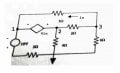# Help me (nodal analysis)

#### MrAl

Joined Jun 17, 2014
10,070
Hi,

Is that a dependent voltage source? Do you know how to handle dependent voltage sources?
Also we might ask is there a 'dot' missing just above the ground symbol or is that really not an actual connection?
If there is no dot there then the 4 Ohm resistor is the only component that connects to ground.

Here is a better drawing with the node numbers redrawn...Last edited:

#### memristor17

Joined Jan 21, 2020
4
Is that a dependent voltage source? Do you know how to handle dependent voltage sources?
hi,
yes,that is a voltage source.
no,i don't know#### MrAl

Joined Jun 17, 2014
10,070
hi,
yes,that is a voltage source.
no,i don't knowHi,

Ok then i assume that you know that ie=(v3-v1)/5 and that is part of the nodal analysis.
So just replace the "4ie" source with a constant source say V4, do the analysis equations, then replace that V4 with 4*ie and try to solve the set of equations then.

#### memristor17

Joined Jan 21, 2020
4
Hi,

Ok then i assume that you know that ie=(v3-v1)/5 and that is part of the nodal analysis.
So just replace the "4ie" source with a constant source say V4, do the analysis equations, then replace that V4 with 4*ie and try to solve the set of equations then.
which circuit program can I solve this question ,do you know?

#### MrAl

Joined Jun 17, 2014
10,070
Hi,

You mean you want to do a simulation or calculate the results yourself using nodal?
I think you should do both really.

There is a free simulator LT Spice you can download. Do a search it will show up quick.

#### memristor17

Joined Jan 21, 2020
4
Hi,

You mean you want to do a simulation or calculate the results yourself using nodal?
I think you should do both really.

There is a free simulator LT Spice you can download. Do a search it will show up quick.
I want to do both

#### WBahn

Joined Mar 31, 2012
28,469
I cannot solve this node analysis question. I think the circuit needs to be solved by redrawing it but I can't figure out how I can solve that method and how can I learn if I have a method like that. Sorry for english.This is google translation. Question:Find node voltages View attachment 198585
The first thing to do is patch up the sloppiness of the person that came up with the problem.

They are claiming that the voltage of the depend source is four times a current. That's like saying that the pressure at the bottom of a tank of water is four times its volume -- it's a fundamentally meaningless statement. In this case it's a fundamentally meaningless statement written by someone that is used to ignoring units and just taking onto the end of the work the units they fervently want the answer to have, regardless of how they might actually work out, and are expecting the people that work the problem to be just as sloppy as they are. So be different, recognize their sloppiness for what it is, and make explicit the assumption you are having to make about what they meant but were too sloppy to state.

In this case, they almost certainly meant that the gain of the dependent source is 4 volts/ampere, or 4 Ω. This is way this particular type of dependent source is known as a transimpedance amplifier.

The traditional way to work this problem is with what is known as a "supernode" equation. So look that up and ponder how it applies to this circuit.

Another way to approach it is to remember that nodal analysis is just a formalized way of applying KCL to each essential node in the circuit where the branch currents are simply written in terms of the node voltages. The problem that this circuit presents is that it's not possible to write the current through the dependent source in that way. So just write an arbitrary symbolic current in that branch and use it. Now combine the two node equations on each side of the dependent source in such a way as to eliminate that current. But now you need another equation but you have one staring you in the face -- that dependent voltage source forces a relationship between the two nodes it's connected to, so use that.

Do the best you can to come up with the three equations you need (you'll actually start with four or even five, but they reduce very quickly to three) and we'll help clear up any problem you have.